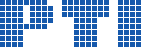Polish Information Processing Society# A hypothetical way to compute an upper bound for the heights of solutions of a Diophantine equation with a finite number of solutions

## Apoloniusz Tyszka

Citation: Proceedings of the 2015 Federated Conference on Computer Science and Information Systems, M. Ganzha, L. Maciaszek, M. Paprzycki (eds). ACSIS, Vol. 5, pages 709716 ()

Full text

Abstract. Let \begin{displaymath} f(n)=\left\{ \begin{array}{ccl} 1 \& {\rm if} \& n=1 \\ 2^{\textstyle 2^{n-2}} \& {\rm if} \& n \in \{2,3,4,5\} \\ \left(2+2^{\textstyle 2^{n-4}}\right)^{\textstyle 2^{n-4}} \& {\rm if} \& n \in \{6,7,8,\ldots\} \end{array} \right. \end{displaymath} \vskip 0.01truecm \noindent We conjecture that if a system $T \subseteq \{x\_i+1=x\_k,~x\_i \cdot x\_j=x\_k:~i,j,k \in \{1,\ldots,n\}\}$ has only finitely many solutions in positive integers \mbox{$x\_1,\ldots,x\_n$}, then each such solution \mbox{$(x\_1,\ldots,x\_n)$} satisfies \mbox{$x\_1,\ldots,x\_n \leqslant f(n)$}. We prove that the function $f$ cannot be decreased and the conjecture implies that there is an algorithm which takes as input a Diophantine equation, returns an integer, and this integer is greater than the heights of integer (\mbox{non-negative} integer, positive integer, rational) solutions, if the solution set is finite. We show that if the conjecture is true, then this can be partially confirmed by the execution of a \mbox{brute-force} algorithm.